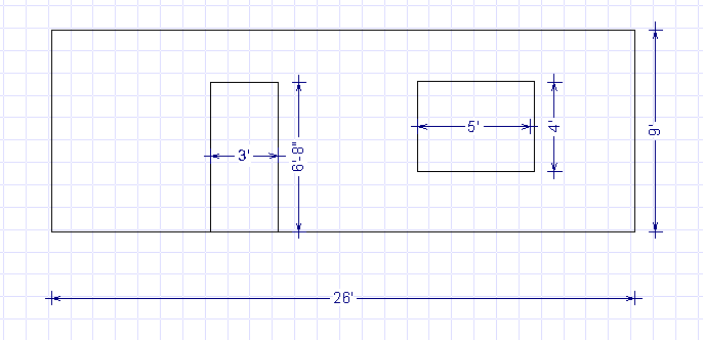Spike's Calculators

# Calculate the Amount of Siding for a Wall

To calculate the amount of siding you will need to cover a wall, you need to know the square footage of the wall, and if you are planning to deduct for openings in the wall, the size of windows and doors. If there are only a few smaller openings in the wall, then ignore them. You more than likely waste the same amount of material as you are saving.##### Calculating the square footage of wall minus openings;
```wall length = 26 feet
wall height = 9 feet
window = 5 feet x 4 feet
door = 3 feet x 6 feet and 8 inches
The siding we are using here is a Fibre-cement board, 144 inches long and 8 1/4-inch wide. We will install it with a 1 1/4-inch overlap, giving it an exposed face of seven inches.
```

To calculate your wall's square footage, take the length 26 feet and the height 9 feet of the wall and multiply these by each other.
`26 * 9  = 234`
`Your wall has a total area of 234 square feet.`

##### Calculate the square footage of your wall openings;
`5 * 4 = 20`
`The window opening is 20 square feet.`

converting the mixed units of feet and inches from the door to inches; 3 feet = 36 inches. 6 feet 8 inches = 80 inches
`36 * 80 = 2880`

divide 2880 by 144 to convert the square inches to square feet (144 square inches in one square foot)
`2880 ÷ 144 = 20`
`The door opening is 20 square feet.`

`Total openings equal 20 + 20 = 40 square feet.`

Subtract the area of the total opening from the walls square footage;
`234 - 40 = 194`
`There are 194 square feet of wall area you have to cover with siding.`

Lineal foot factor; Calculate the lineal foot factor of your siding. To calculate the factor, take the exposed face value of your siding and divide it by 12; (this calculates the number of rows in 12 inches)
`12 ÷ 7 = 1.743`
`This siding has a lineal foot factor of 1.743.`

##### Calculate the amount of siding needed for your wall;
Multiply the square footage of the wall of 72 square feet by 1.743. The result is the number of boards in lineal feet;
`194 * 1.743 = 338.142 rounded to 339`
`You will need 339 lineal feet of siding`

##### Convert the lineal feet to the number of boards;
144 inches = 12 feet. The boards are 12 feet long. Divide the lineal feet amount by 12 to get the number of boards needed;
`339 ÷ 12 = 28.25 rounded to 29`
`You will need 29 boards of siding to cover the wall.`

This amount does not allow for any waste!